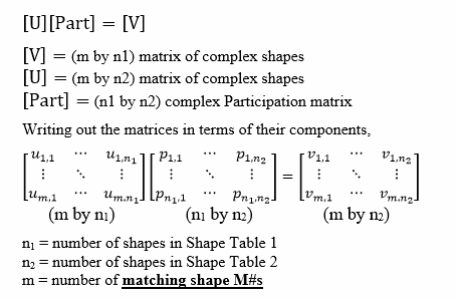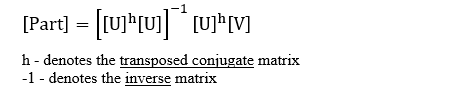# Tools | Participation Matrix

Calculates and displays the shape Participation Matrix.

The Participation Matrix equates the shapes in one Shape Table with the shapes of another Shape Table.

• Each Shape Table can contain ODS's, FEA Mode Shapes, EMA Mode Shapes, or Engineering Data Shapes.

Two sets of complex valued shapes can be assembled into two matrices [U] & [V], where each column of each matrix contains a shape. The two shape matrices are equated to each other with the following matrix equation,## Least-Squared-Error Solution

The Participation Matrix is computed as a least-squared-error solution to the above equation using the formula below.The two shape matrices [U] & [V] must have some matching DOFs (shape components)
If the shapes [U] are linearly independent of one another for the matching DOFs, the Participation Matrix can be computed
Only mode shapes
are used to calculate the Participation Matrix. Modal Frequency & Damping are not used.